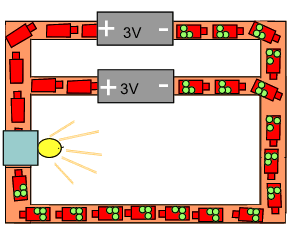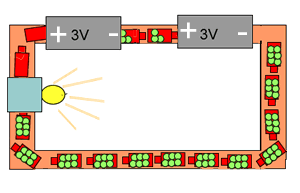Battery connections in series and parallel To explain what happens when batteries are connected in certain ways we will think of electrons as little trucks carrying bags of energy. These bags of energy we will call voltage. As the trucks pass through the batteries they get a certain amount of energy depending on the battery. The trucks travel around the circuit giving up all their energy to appliances that they pass through. Lets apply our analogy to the circuits below, Batteries can be connected as shown on the right. This type of circuit is called a parallel circuit. Notice how each battery puts out trucks with 3 bags of energy. Each truck delivers 3 bags of energy to the light globe.How many bags of energy does the light globe receive? Now consider the circuit on the right. This is a series circuit. Notice how many volts each electron has when passing through the light globe as compared to the circuit above.1) What is happening to the amount of energy given to each electron as it passes through successive batteries? increases decreases remains unchanged 2) Will the light globe glow brighter in this circuit? Explain. 3) A 3 volt and a 9 volt battery are connected in series. A 12 volt toy is connected to the circuit. Will the toy work? 4) A 3 volt battery is connected to a circuit. Another 3 volt battery is connected to the circuit in series. After the connection of the second battery the a) current should increases decreases remains unchanged b) voltage should increases decreases remains unchanged 5) A 3 volt battery is connected to a circuit. Another 3 volt battery is connected to the circuit in parallel. After the connection of the second battery the a) current should increases decreases remains unchanged b) voltage should increases decreases remains unchanged 6) Voltage can be described as the the amount of electrons flowing in a circuit electron traveling in a circuit energy or push given to an electron 7) Current is the amount of electrons flowing in a circuit electron traveling in a circuit energy or push given to an electron 8) A 6v battery is connected to a 6 v light globe. What is the voltage of the electrons as they return back to the battery? 0 6 12 9 Exercises Continue